# Texas Go Math Grade 8 Lesson 11.1 Answer Key Equations with the Variable on Both Sides

Refer to our Texas Go Math Grade 8 Answer Key Pdf to score good marks in the exams. Test yourself by practicing the problems from Texas Go Math Grade 8 Lesson 11.1 Answer Key Equations with the Variable on Both Sides.

## Texas Go Math Grade 8 Lesson 11.1 Answer Key Equations with the Variable on Both Sides

Explore Activity

Modeling an Equation with a Variable on Both Sides

Algebra tiles can model equations with a variable on both sides.

Use algebra tiles to model and solve x + 5 = 3x – 1.Reflect

Question 1.
How can you check the solution to x + 5 = 3x – 1 using algebra tiles?
The following model for the equation x + 5 = 3x – 1 is given. We found on the previous exercise that the solution was x = 3 and now we need to check the solution using algebra tiles.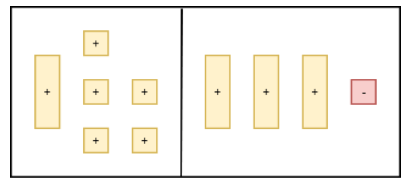Place one +1-tile on both sides and remove the zero pairsReplace every x-tile with three of +1-tiles. We can see that the number of +1-tiles is equal on both sides, therefore the solution that we had found proves the equation.Example 1

Andy’s Rental Car charges an initial fee of $20 plus an additional$30 per day to rent a car. Buddy’s Rental Car charges an initial fee of $36 plus an additional$28 per day. For what number of days is the total cost charged by the companies the same?
STEP 1: Write an expression representing the total cost of renting a car from Andy’s Rental Car.
Initial fee + Cost for x days
20 + 30x

STEP 2: Write an expression representing the total cost of renting a car from Buddy’s Rental Car.
Initial fee + Cost for x days
36 + 28x

STEP 3: Write an equation that can be solved to find the number of days for which the total cost charged by the companies would be the same.
Total cost at Andy’s = Total cost at Buddy’s
20 + 30x = 36 + 28x

STEP 4: Solve the equation for x.The total cost is the same if the rental is for 8 days.

Question 2.
A water tank holds 256 gallons but is leaking at a rate of 3 gallons per week. A second water tank holds 384 gallons but is leaking at a rate of 5 gallons per week. After how many weeks will the amount of water in the two tanks be the same?
Write an expression for the remaining gallons in the first tank.
A water tank holds 256 gallons 3 gallons per x weeks:
256 – 3x
Write an expression for the remaining gallons in the second tank.
A second water tank holds 384 gallons – 5 gallons per x weeks:
384 – 5x
Write an equation that can be solved to find the number of weeks for which the amount of water in the two tanks would be the same.
The remaining gallons in the first tank = the remaining gallons in the second tank:
256 – 3x = 384 – 5x
Solve the equation for x
(Write the equation.)256 – 3x = 384 – 5x
(Add 5x to both sides.)256 – 3x + 5x = 384 – 5x + 5x
256 + 2x = 384
(Subtract 256 from both sides.)256 + 2x – 256 = 384 — 256
2x = 128
(Divide both sides by 2) $$\frac{2x}{2}$$ = $$\frac{128}{2}$$
x = 64
The amount of water in the two tanks will be the same for 64 weeks.

Example 2

Write a real-world situation that could be modeled by the equation 150 + 25x = 55x.
STEP 1: The left side of the equation consists of a constant plus a variable term. It could represent the total cost for doing a job where there is an initial fee plus an hourly charge.

STEP 2: The right side of the equation consists of a variable term. It could represent the cost for doing the same job based on an hourly charge only.

STEP 3: The equation 150 + 25x = 55x could be represented by this situation: A handyman charges $150 plus$25 per hour for house painting. A painter charges $55 per hour. How many hours would a job have to take for the handyman’s fee and the painter’s fee to be the same? Question 3. Write a real-world situation that could be modeled by the equation 30x = 48 + 22x. Answer: Ben’s Rental Car charges just 30 dollars per day to rent a car. Marry’s Rental Car charges an initial fee of 48 dollars plus an additional 22 dollars per day. For what number of days is the total cost charged by the companies the same? Texas Go Math Grade 8 Lesson 11.1 Guided Practice Answer Key Use algebra tiles to model and solve each equation. (Explore Activity) Question 1. x + 4 = -x – 4 _____________ Answer: Model x + 4 on the left side of the mat and -x – 4 on the right side.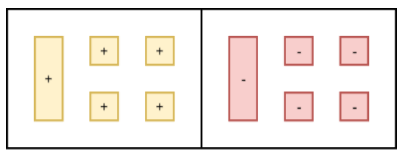Add one x-tile to both sides. This represents adding x to both sides of the equation. Remove zero pairs.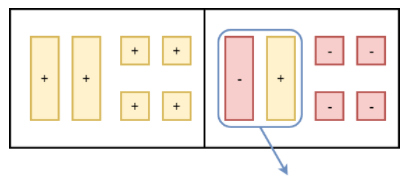Place four -1-tiles on both sides. This represents subtracting -4 from both sides of the equation. Remove zero pairs.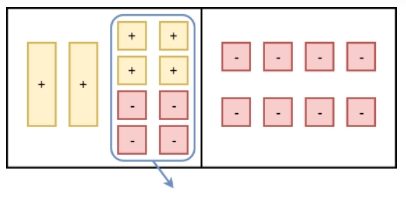Separate each side into 2 equal groups. One x-tile is equivalent to four -1 -tiles.The solution is x = -4 Question 2. 2 – 3x = -x – 8 _____________ Answer: Solve the equation for x. 2 – 3x = -x – 8 (Add 3 to both sides)2 – 3x + 3x = -x – 8 + 3x 2 = 2x – 8 (Add 8 to both sides.)2 + 8 = 2x – 8 + 8 10 = 2x or 2x = 10 (Divide both sides by 2)$$\frac{2x}{2}$$ = $$\frac{10}{2}$$ x = 5 Check: 2 – 3(5) = -5 – 8 2 – 15 = -13 (for x = 5) -13 = -13 x = 5 Question 3. At Silver Gym, membership is$25 per month, and personal training sessions are $30 each. At Fit Factor, membership is$65 per month, and personal training sessions are $20 each. In one month, how many personal training sessions would Sarah have to buy to make the total cost at the two gyms equal? (Example 1) Answer: Write an equation for the membership at Silver Gym Membership + Personal training session 25 + 30x Write an equation for the membership at Fit Factor Membership + Personal training session 65 + 20x Write an equation that can be solved to find the number of weeks for which the number of gallons in the two tank would be the same Membership at Silver Gym = Membership at Fit Factor 25 + 30x = 65 + 20x Solve for x 25 + 30x = 65+20x Subtract 20x from both sides 25 + 30x – 20x = 65 + 20x – 20x 25 + 10x = 65 Subtract 25 from both sides 25 + 10x – 25 = 65 – 25 10x = 40 Divide both sides by 10 x = $$\frac{40}{10}$$ = 4 Sarah would have to buy$4$session for the total cost at the two gyms to be equal. Question 4. Write a real-world situation that could be modeled by the equation 120 + 25x = 45x. (Example 2) Answer: Given 120 + 25x = 45x The Left side of the equation consists of a constant plus a variable Sarah offers a plan to tutor a student at$25 per her plus a one-time registration fee of $120. The right side of the equation consists of a variable term. Surah offers an alternative plan to tutot a student at$45 per hour and no registration fee.

How many hours would a student have to take for the two plans cost the same
120 + 25x = 45x

Question 5.
Write a real-world situation that could be modeled by the equation 100 – 6x = 160 – 10x. (Example 2)
A water tanks holds 100 gallons but is leaking at a rate of 6 gallons per week. A second water tank holds 160 gallons but is leaking at a rate of 10 gallons per week. After how many weeks will the amount of water in the two tanks be the same?

Essential Question Check-In

Question 6.
How can you solve an equation with the variable on both sides?
Isolate the variable on one side Add/subtract the variable with a lower coefficient from both sides Add/subtract the constant (with the variable) from both sides. Divide both sides by coefficient of isolated variable.

Question 7.
Derrick’s Dog Sitting and Darlene’s Dog Sitting are competing for new business. The companies ran the ads shown.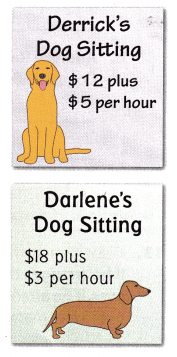a. Write and solve an equation to find the number of hours for which the total cost will be the same for the two services.
Write an expression for the cost of Derrick’s Dog Sitting.
5x + 12
Write an expression for the cost of Darlenes Dog Sitting.
3x + 18
Write an equation that can be solved to find the number of hours for which the cost would be the same.
5x + 12 = 3x + 18
Solve the equation for x
(Write the equation.)5x + 12 = 3x + 18
(Subtract 3x from both sides.)5x + 12 – 3x = 3x + 18 – 3x
2x + 12 = 18
(Subtract 12 from both sides.)2x + 12 – 12 = 18 – 12
2x = 6
(Divide both sides by 2)$$\frac{2x}{2}$$ = $$\frac{6}{2}$$
x = 3

b. Analyze Relationships Which dog sitting service is more economical to use if you need 5 hours of service? Explain.
The total cost will. be the same for 3 hours.
The unknown x is 5 hours
5(5) + 12 = 25 + 12 = 37
3(5) + 18 = 15 + 18 = 33
33 < 37
Darlene’s Dog Sitting would be cheaper.

Question 8.
Country Carpets charges $22 per square yard for carpeting, and an additional installation fee of$100. City Carpets charges $25 per square yard for the same carpeting, and an additional installation fee of$70.
a. Write and solve an equation to find the number of square yards of carpeting for which the total cost charged by the two companies will be the same.
Country Carpets charges 22 dollars per square yard for carpeting, and an additional installation fee of 100 dollars.
Write an expression:
22x + 100
City Carpets charges 25 dollars per square yard for the same carpeting, and an additional installation fee of 70 dollars.
Write an expression:
25x + 70
Write and solve an equation to find the number of square yards of carpeting for which the total cost charged by the two companies will be the same.
22x + 100 = 25x + 70
Solve the equation for x.
(Write the equation.)22x + 100 = 25x + 70
(Subtract 22x from both sides.)22x + 100 – 22x = 25x + 70 – 22x
100 = 3x + 70
(Subtract 70 from both sides.) 100 – 70 = 3x + 70 – 70
30 = 3x
(Divide both sides by 3.) $$\frac{3x}{3}$$ = $$\frac{30}{3}$$
x = 10
The total cost will be the same for 10 square yards of carpeting.

b. Justify Reasoning Mr. Shu wants to hire one of the two carpet companies to install carpeting in his basement. Is he more likely to hire Country Carpets or City Carpets? Explain your reasoning.
x < 10
22(9) + 100 = 198 + 100 = 298
25(9) + 70 = 225 + 70 = 295
City Carpets are cheaper when x < 10. x > 10
22(11) + 100 = 232 + 100 = 342
25(11) + 70 = 275 + 70 = 345
Country Carpets are cheaper when x > 10.
If Mr. Shu needs the carpeting done for less than 10 square yards, he will hire City Carpets and if he needs carpeting for more than 10 square yards, he will hire Country Carpets.

Write an equation to represent each relationship. Then solve the equation.

Question 9.
Two less than 3 times a number ¡s the same as the number plus 10.
Given
Two less than 3 times a number is the same as the number plus 10
Represent the unknown with a variable Times means multiplication
Two less than 3 times x is the same as x plus 10
Two less than 3x is the same as x + 10
Less than means subtraction
3x – 2 is the same as x + 10
Place the equal sign
3x – 2 = x + 10
Solve for x
3x – 2 = x + 10
Subtract x from both sides
3x – 2 – x = x + 10 – x
2x – 2 = 10
2x – 2 + 2 = 10 + 2
2x = 12
Divide both sides by 2
x = $$\frac{12}{2}$$ = 6
x = 6

Question 10.
A number increased by 4 is the same as 19 minus 2 times the number.
Given
A number increased by 4 is the same as 19 minus 2 times the number
Represent the unknown with a variable Times means multiplication
x increased by 4 is the same as 19 minus 2x
x + 4 is the same as 19 minus 2x
minus means subtraction
x + 4 is the same as 19 – 2x
Place the equal sign
x + 4 = 19 – 2x
Solve for x
x + 4 = 19 – 2x
x + 4 + 2x = 19 – 2x + 2x
3x + 4 = 19
Subtract 4 from both sides
3x + 4 – 4 = 19 – 4
3x = 15
Divide both sides by 3
x = $$\frac{15}{3}$$ = 5

Question 11.
Twenty less than 8 times a number is the same as 15 more than the number.
Given
Twenty less than 8 times a number is the same as 15 more than the number
Represent the unknown with a variable Times means multiplication
Twenty less than 8 times x is the same as 15 more than the x
Twenty less than 8x is the same as x + 15
Less than means subtraction
8x – 20 is the same as x + 15
Place the equal sign
8x – 20 = x + 15
Solve for x
8x – 20 = x + 15
Subtract x from both sides
8x – 20 – x = x + 15 – x
7x – 20 = 15
7x – 20 + 20 = 15 + 20
7x = 35
Divide both sides by 7
x = $$\frac{35}{7}$$ = 5

Question 12.
The charges for an international call made using the calling card for two phone companies are shown in the table.a. What is the length of a phone call that would cost the same no matter which company is used?
The charges for an international call made using the calling card for Company A.
35 + 3x
The charges for an internationaL call made using the calling card for Company B.
45 + 2x
Write an equation that can be solved to find the length of a phone call that would cost the same no matter which company is used.
35 + 3x = 45 + 2x
Solve the equation for x.
(Write the equation.) 35 + 3x = 45 – 2x
(Subtract 2x from both sides.) 35 + 3x – 2x = 45 + 2x – 2x
35 + x = 45
(Subtract 35 from both sides.) 35 + x – 35 = 45 – 35
x = 10

b. Analyze Relationships When is it better to use the card from Company B?
A phone call would cost the same, no matter which company is used, for 10 minutes.
Check solution if x > 10, for example, it can be x = 11:
(Company A) 35 + 3(11) = 35 + 33 = 68 dollars
(Company B) 45 + 2(11) = 45 + 22 = 67 dollars
If the length of the phone call is more then 10 minutes, it’s cheaper to use the card from Company B.

H.O.T. Focus on Higher Order Thinking

Question 13.
Draw Conclusions Liam is setting up folding chairs for a meeting. If he arranges the chairs in 9 rows of the same length, he has 3 chairs left over. If he arranges the chairs in 7 rows of that same length, he has 19 left over. How many chairs does Liam have?
The number of chairs in 9 rows and 3 chairs left over:
9 + 3
The number of chairs in 7 rows and 19 chairs left over
7x + 19
Write an equation that can be solved to find the number of chairs in a row for which the total number of chairs is the same
9x + 3 = 7x + 19
Solve the equation for x
(Write the equation) 9x + 3 = 7x + 19
(Subtract 7x from both sides) 9x + 3 – 7x = 7x + 19 – 7x
2x + 3 = 19
(Subtract 3 from both sides) 2x + 3 – 3 = 19 – 3
2x = 16
(Divide both sides by 2) $$\frac{2x}{2}$$ = $$\frac{16}{2}$$
x = 8
The total number of chairs is:
9(8) + 3 = 72 + 3 = 75
75 chairs

Question 14.
Explain the Error Rent-A-Tent rents party tents for a flat fee of $365 plus$125 a day. Capital Rentals rents party tents for a flat fee of $250 plus$175 a day. Delia wrote the following equation to find the number of days for which the total cost charged by the two companies would be the same:
365x + 125 = 250x + 175
Find and explain the error in Delia’s work. Then write the correct equation.
The error is that she attached the variable with the flat fee (which is constant) and put the daily rent as a constant (which is variable).
The correct equation 5:
365 + 125x = 250 + 175x

Question 15.
Persevere in Problem Solving Lilliana is training for a marathon. She runs the same distance every day for a week. On Monday, Wednesday, and Friday, she runs 3 laps on a running trail and then runs 6 more miles. On Tuesday and Sunday, she runs 5 laps on the trail and then runs 2 more miles. On Saturday, she just runs laps. How many laps does Lilliana run on Saturday?
Let x be the number of miLes for one lap.
On Monday, Wednesday and Friday, Lituana runs 3 Laps on a running trail and then runs 6 more miles.
Write an expression:
3x + 6
On Tuesday and Sunday, she runs 5 laps on the trail and then runs 2 more miles.
Write an expression:
5x + 2
She runs the same distance every day for a week.
3x + 6 = 5x + 2
Solve the equation for x.
(Write the equation.) 3x + 6 = 5x + 2
(Subtract 3x from both sides.) 3x + 6 – 3x = 5x + 2 – 3x
6 = 2x + 2
(Subtract 2 from both sides.) 6 – 2 = 2x + 2 – 2
4 = 2x
(Divide both sides by 2.) $$\frac{2x}{2}$$ = $$\frac{4}{2}$$
x = 2
Lulliana runs 2 miles for one lap.
The number of miLes she runs every day for a week:
5(2) + 2 = 10 + 2 = 12 laps
2 miles for one lap:
$$\frac{12}{2}$$ = 6 laps on Saturday.

Scroll to Top